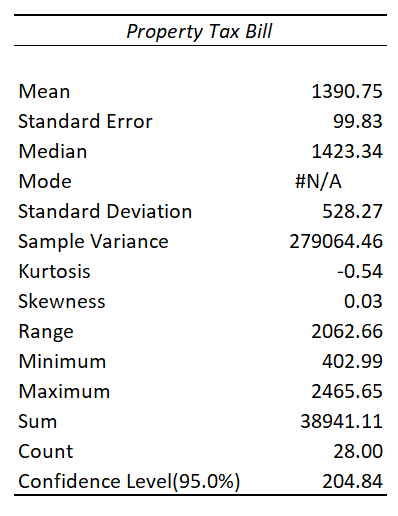# A tax assessor wants to assess the mean property tax bill for all homeowners in a certain state....

## Question:

A tax assessor wants to assess the mean property tax bill for all homeowners in a certain state. From a survey ten years ago, a sample of 28 property tax bills is given below. Assume the property tax bills are approximately normally distributed. Use Excel to construct a 95% confidence interval for the population mean property tax bill. Round your answers to two decimal places and use increasing order.

Property Tax Bill
1715.35
1157.82
651.10
1093.36
1609.22
1391.47
1398.89
402.99
1028.76
1571.23
529.42
1579.48
699.56
2222.16
838.60
866.41
1066.60
1498.64
1784.28
2016.99
2465.65
1098.81
1346.65
1574.00
1447.78
2018.33
2156.75
1710.81

## Confidence interval

The confidence interval is the type of estimation for the population parameters, and it provides the range which includes the lower limit and upper limit for the values for population parameters (population mean, population standard deviation, and other parameters)

Given Information

Sample size; 28

The steps for the 95% confidence interval are obtained from the excel is given as;

-Go to the Data tab in the ribbon and select a data analysis tool.

-Select descriptive statistics and select input range which is list data.

-choose the output range and check the summary statistics and confidence interval for the mean.

The output range for the data is given as;The lower limit and upper limit for mean is given as;

{eq}\begin{align*} {\rm{lower \ limit}} &= 1390.75 - 204.84\\ &= 1185.91 \end{align*} {/eq}

{eq}\begin{align*} {\rm{Upper \ limit}} &= 1390.75 - 204.84\\ &= 1595.59 \end{align*}{/eq}

Therefore, the 95% confidence interval is (1185.91, 1595.59).